# Organic Chemistry A Short Course

Chemistry

## Quiz 7 :Alcohols, Phenols ThiolsLooking for Organic Chemistry Homework Help?

## Quiz 7 :Alcohols, Phenols Thiols

Showing 1 - 20 of 32Which of the following is a secondary (2°) alcohol?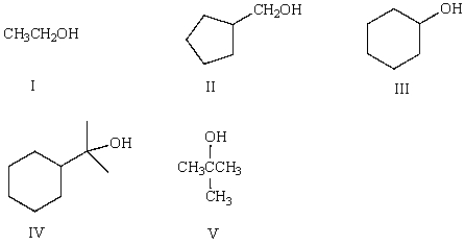Free
Multiple Choice

CWhich of the following is 3-pentyn-1-ol?
Free
Multiple Choice

CWhat is the IUPAC name for isobutyl alcohol?
Free
Multiple Choice

DWhat would be the IUPAC name for the following alcohol?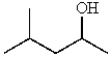Multiple ChoiceWhich of the following molecules is classified as a tertiary (3°) alcohol?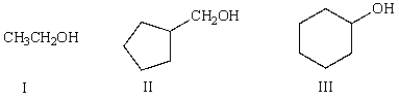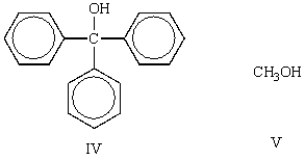Multiple ChoiceWhat is the correct name for the following molecule?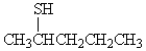Multiple ChoiceWhat is the name of the following alcohol?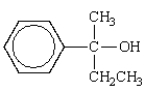Multiple ChoiceWhat is a correct name for (CH3)3CO- Na+??
Multiple ChoiceThe correct name for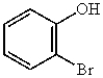is
Multiple ChoiceThe formula for 2-pentanethiol is:
Multiple ChoiceWhich of the following molecules would be the best hydrogen bond donor?
Multiple ChoiceWhich of the following molecules would have the highest boiling point?
Multiple ChoiceThe expected order of boiling points of the following is: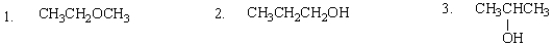Multiple ChoiceA Lewis acid is a:
Multiple ChoiceThe conjugate base of carbonic acid, H2CO3, is:
Multiple ChoiceWhich of the following molecules would be the strongest Brønsted-Lowry acid?
Multiple ChoiceWhich of the following phenols is the strongest acid?
Multiple ChoiceWhich of the following is the strongest base?
Multiple Choice